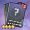# 对抗模拟指令

2023-08-31更新

## 基础数据

• 对抗模拟指令
• 耐久
•  初始 200
•  强化+10 500
•  强化+11 530
• 大型作战塞壬增伤 强化+11后获得此属性
• 强化+11 1%
• 技能 对抗模拟指令
• 描述 装备时，每15秒触发一种随机指令效果
• 装备参数
• 所属阵营 其他
• 适用舰种
驱逐
轻巡
重巡
超巡
战巡
战列
轻航
航母
航战
潜母
重炮
潜艇
维修
运输
风帆S
风帆V
风帆M

整装获取 其他：信息对抗模拟测试(1) 为自身生成可抵消最大耐久3%的护盾，持续8秒。
(2) 回复自身最大耐久2%。
(3) 发射雷击弹幕，30×4hit磁性鱼雷，对甲补正80% 100% 130%，人形锁定。
(4) 发射炮击弹幕，10×16hit通常弹，对甲补正120% 120% 120%，人形锁定。## 装备导航

鱼雷 • 星云舞裙  • 引力舞鞋  • 闪耀之歌  • 活力之歌  • 炽烈之歌  • 改良声呐  • 对抗模拟指令  • 基础声呐  • 维修工具  • 陀螺仪  • 改良锅炉  • 灭火器  • 海军迷彩  • 液压舵机
舰炮 • 超重弹  • 珍珠之泪  • SG雷达  • 雪顶之梦  • 火控雷达  • 对空雷达
舰炮 • 海大黄蜂  • 海怒  • 海毒牙  • 海喷火  • 海飓风  • 海斗士 • 飞龙  • 火冠  • 火把  • 梭鱼  • 青花鱼  • 剑鱼 • 萤火虫  • 海燕  • 贼鸥 • 小王冠  • 归航信标 • 刺猬弹
舰炮 • 紫电改二  • 烈风 • 流星  • 天山改  • 天山 • 彗星 • Z旗  • 刺绣锦囊 • 晴岚  • 强风  • 瑞云
舰炮 • 梅之语  • 四神之印 • 上游-1
舰炮 • 海魂迷彩
舰炮 • 天使之羽
舰炮 • 飓风旗  • 帆索组件
设备 • 智慧模块  • 晃悠悠
设备 • 鮟鱇肝  • 玉米灯笼  • 组徽
设备 • 心之钥匙  • 觉醒宝珠
设备 • 偶像手环
设备 • 煌翼炎龙  • 征战巨坦 • 木龙雕塑
设备 • 形意口琴  • 泡云弹车  • 创世之槌  • Ｎ／Ａ  • 默示录  • 万灵药剂  • 涡旋风精  • 震耳雷球  • 结晶冰精  • 炙烈炎烧
设备 • 忍术卷轴  • 忍者装束
通常 • 苦无  • 短剑  • 权杖  • 猎弓  • 轻弩  • 指挥刀  • 骑枪  • 大剑  • 手弩  • 铁剑  • 单手锤  • 双剑 • 莱伯君  • 全开标枪  • 摇曳悠然 • 幻想之环  • 皮罗什基 • 光风霁月 • 魅力四射  • 不再哭泣 • 鸾凤流丽 • 一张字条 • 烤白薯  • 马卡龙  • 帕帕纳西  • 甜馅馒头  • 公主蛋糕 • 监禁程序  • 龙的饰品  • 演出棒  • 怪兽种子  • 戴拿飞翼 • 百合长老  • 百花盛开  • 幽兰爪  • 爱瑟尔剑  • 暗夜星矢  • 闪耀梦想 • 太刀・钢